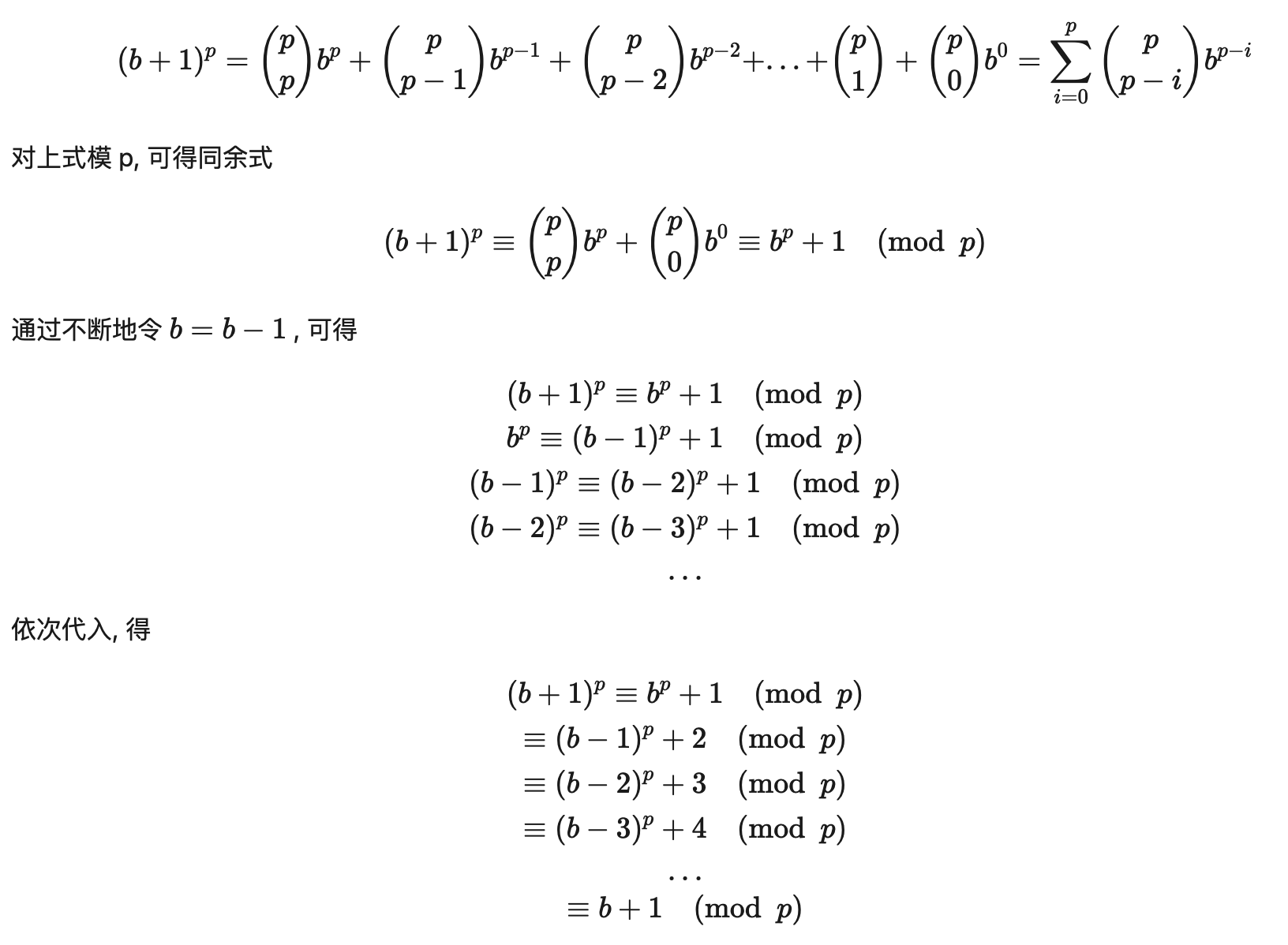# 你真的了解 RSA 加密算法吗？## 一、什么是素数

boolean isPrime = number > 0;
// 计算number的平方根为k，可以减少一半的计算量
int k = (int) Math.sqrt(number);
for (int i = 2; i 

## 二、对称加密和非对称加密## 三、算法公式推导• 5相当于 Alice 要传递给 Bob 的信息
• 3相当于是秘钥
• 6相当于是加密后的信息

5^1 mod 7 = 5
5^2 mod 7 = 3
5^3 mod 7 = 6
5^4 mod 7 = 2
...## 四、关于RSA算法

RSA加密算法是一种非对称加密算法，在公开秘钥加密和电子商业中被广泛使用。1973年，在英国政府通讯总部工作的数学家克利福德·柯克斯（Clifford Cocks）在一个内部文件中提出了一个与之等效的算法，但该算法被列入机密，直到1997年才得到公开。

RSA 的算法核心在于取了2个素数做乘积求和、欧拉计算等一系列方式算得公钥和私钥，但想通过公钥和加密信息，反推出来私钥就会非常复杂，因为这是相当于对极大整数的因数分解。所以秘钥越长做因数分解越困难，这也就决定了 RSA 算法的可靠性。—— PS：可能以上这段话还不是很好理解，程序员👨🏻‍💻还是要看代码才能悟。接下来我们就来编写一下 RSA 加密代码。

## 五、实现RSA算法

RSA 的秘钥生成首先需要两个质数p、q，之后根据这两个质数算出公钥和私钥，在根据公钥来对要传递的信息进行加密。接下来我们就要代码实现一下 RSA 算法，读者也可以根据代码的调试去反向理解 RSA 的算法过程，一般这样的学习方式更有抓手的感觉。嘿嘿 抓手

### 2. 乘积n

n = p * q 的乘积。

public long n(long p, long q) {
return p * q;
}


### 3. 欧拉公式 φ(n)

φ(n) = (p - 1) * (q - 1)

public long euler(long p, long q) {
return (p - 1) * (q - 1);
}


### 4. 选取公钥e

e 的值范围在 1

public long e(long euler){
long e = euler / 10;
while (gcd(e, euler) != 1){
e ++;
}
return e;
}


### 5. 选取私钥d

d = (kφ(n) + 1) / e

public long inverse(long e, long euler) {
return (euler + 1) / e;
}


### 6. 加密

c = m^e mod n

public long encrypt(long m, long e, long n) {
BigInteger bM = new BigInteger(String.valueOf(m));
BigInteger bE = new BigInteger(String.valueOf(e));
BigInteger bN = new BigInteger(String.valueOf(n));
return Long.parseLong(bM.modPow(bE, bN).toString());
}


### 7. 解密

m = c^d mod n

public long decrypt(long c, long d, long n) {
BigInteger bC = new BigInteger(String.valueOf(c));
BigInteger bD = new BigInteger(String.valueOf(d));
BigInteger bN = new BigInteger(String.valueOf(n));
return Long.parseLong(bC.modPow(bD, bN).toString());
}


### 8. 测试

@Test
public void test_rsa() {
RSA rsa = new RSA();
long p = 3,                         // 选取2个互为质数的p、q
q = 11,                     // 选取2个互为质数的p、q
n = rsa.n(p, q),            // n = p * q
euler = rsa.euler(p, q),    // euler = (p-1)*(q-1)
e = rsa.e(euler),           // 互为素数的小整数e | 1 

消息：5


• 通过选取3、11作为两个互质数，计算出公钥和私钥，分别进行消息的加密和解密。如测试结果消息5的加密后的信息是26，解密后获得原始信息5

## 六、RSA数学原理

### 1. 模运算

#### 1.1 整数除法

• 对任意整数 m,n，如果 a∣b 且 a∣c, 则 a∣(mb + nc)
• 如果 a∣b, 则对于所有整数 c 都有 a∣bc
• 如果 a∣b 且 b∣c, 则 a∣c

#### 1.2 模算术

a ≡ b(mod m) 和 a mod m= b 很相似. 事实上, 如果 a mod m = b, 则 a≡b(mod m). 但他们本质上是两个不同的概念. a mod m = b 表达的是一个函数, 而 a≡b(mod m) 表达的是两个整数之间的关系.

(a+b)mod m=((a mod m)+(b mod m)) mod m

ab mod m=(a mod m)(b mod m) mod m

(ac−bc) mod m=c(a−b) mod m=(c mod m⋅(a−b) mod m) mod m=0

∴ ac ≡ bc(mod m)

(ac−bc)mod m=c(a−b)mod m=(c mod m⋅(a−b)mod m) mod m=0

∵ c mod m≠0 ,

∴ (a−b) mod m=0,

∴a ≡ b(mod m) .

### 2. 最大公约数

#### 2.1 求最大公约数

287=91⋅3+14

91=14⋅6+7

14=7⋅2+0

def gcd(a, b):
if b == 0: return a
return gcd(b, a % b)


#### 2.2 贝祖定理∴ r=0 , d0∣a.

∴∴ d0 是 a 和 b 的最大公约数.

def gcd(a, b):
if b == 0: return a, 1, 0
d, x, y = gcd(b, a % b)
return d, y, x - (a / b) * y


### 3. 线性同余方程

∴ x 为 a 模 m 的逆.

a 模 m 的逆也被称为 a 在模m乘法群 Z∗m 中的逆元. 这里我并不想引入群论, 有兴趣的同学可参阅算法导论

x≡¯ab(modm) 就是方程的解. 注意同余方程会有无数个整数解, 所以我们用同余式来表示同余方程的解.### 4. 中国余数定理### 5. 费马小定理### 6. 算法证明

RSA 算法按照以下过程创建公钥和私钥:

1. 随机选取两个大素数 p 和 q, p≠qp≠q;
2. 计算 n=pq
3. 选取一个与 (p−1)(q−1) 互素的小整数 e;
4. 求 e 模 (p−1)(q−1) 的逆, 记作 d;
5. 将 P=(e,n)公开, 是为公钥;
6. 将 S=(d,n)保密, 是为私钥.(1) 式表明, 不仅可以用公钥加密, 私钥解密, 还可以用私钥加密, 公钥解密. 即加密计算 C=M^d mod n, 解密计算 M=C^e mod n

RSA 算法的安全性基于大整数的质因数分解的困难性. 由于目前没有能在多项式时间内对整数作质因数分解的算法, 因此无法在可行的时间内把 n 分解成 p 和 q 的乘积. 因此就无法求得 e 模 (p−1)(q−1)的逆, 也就无法根据公钥计算出私钥.

## 七、常见面试题

• 质数的用途
• RSA 算法描述
• RSA 算法加解密的过程
• RSA 算法使用场景
• 你了解多少关于 RSA 的数学数论知识

THE END